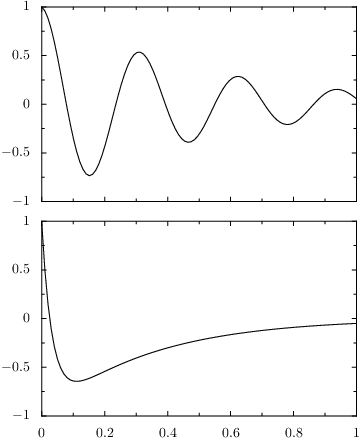0.4 KB
57.5 KB
57.2 KB
27.2 KB
35.6 KB

## Linking one axis to another one```import math
from pyx import *

c = canvas.canvas()

g1 = c.insert(graph.graphxy(width=8))
g1.plot(graph.data.function("y(x)=2*exp(-30*x)-exp(-3*x)", min=0, max=1))

g2 = c.insert(graph.graphxy(width=8, ypos=g1.height+0.5,
g2.plot(graph.data.function("y(x)=cos(20*x)*exp(-2*x)"))

When plotting two or more related graphs, you sometimes want to have identical axes. This example demonstrates how to achieve this goal in PyX using a `linkedaxis`.
In order to arrange the two graphs, we first create a canvas `c`, in which we insert the first graph `g1` (the lower panel in the output), which contains the graph of a function.
In the next graph `g2` (the upper panel in the output), we now refer to the x-axis of `g1`. We do this by creating a `graph.axis.linkedaxis` instance passing the x-axis of `g1` as the only argument. The latter is obtained from the axis dictionary `axes` of the graph.
Note that for a linked axis, PyX automatically turns off the drawing of the axis labels, as you can see in the upper panel. If you do not like this behaviour, you have to pass a different axis painter to the `linkedaxis` constructor.